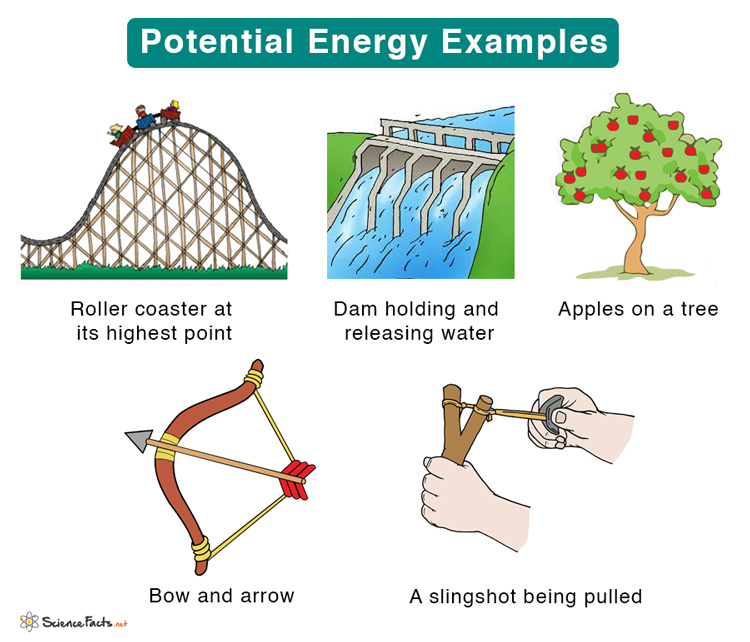# Mechanical energy and It’s types

• The energy possessed by a body because of its motion and position or state is called mechanical energy.
• For example: a moving car possesses energy; a stone lying at height from the ground possesses energy; a compressed or stretched spring possesses energy and so on.
• The mechanical energy is of two types. They are

A) Kinetic energy

• Kinetic energy of a body is defined as the energy possessed by the body by virtue of its motion.
• A walking person, a moving vehicle, a fired bullet, moving water in river all possesses kinetic energy.Image source: javatpoint

Formula of Kinetic Energy

Let us consider a body of mass ‘m is initially at rest. If a force of magnitude ‘F’ is applied on the body, it comes in motion and produces an acceleration ‘a’on the body.

Also let the body travels a distance ‘s’ after sometime and attains a velocity ‘v’.

Here, the force F does work on the body. As the force goes on doing work, it spends energy. The energy thus spent in the form of work done is converted into the kinetic energy of the body.

If the friction between the body and the ground is neglected, the kinetic energy gained by the body is equal to the work done by the force in displacing the body by the distance ‘s’.Image source: howmechanism                             Image source: scienceonline

For the body,

Initial velocity (u) = 0

Final velocity = v

Acceleration = a

Distance travelled = s

We have

v2 = u2 + 2as

Or, v2 = 0 +2as

as = v2/2……………………………. (i)

Now, the work done by the force (W) = force × distance

= F × s

Also, F = mass × acceleration = m × a

∴ W = m×a×s………………………………(ii)

From (i) and (ii), we have

W = mv2/2

Thus, the kinetic energy of a body is given by KE = ½(mv2)

B) Potential energy

• Potential energy of a body is defined as the energy possessed by it due to its position, shape or state.
• A body lying at a height, a stretched catapult and water stored in a dam all possess potential energy.Image source: sciencefacts

Gravitational potential energy

Consider a body of mass ‘m’ lying at rest at a height ‘h’. if the body is allowed to fall freely, its velocity goes on increasing. The body is under the action of the gravity alone. So, the acceleration produced on the body is the ‘g’.

When the body is at the height, it possesses potential energy. When it begins falling down, its potential energy is converted to kinetic energy.

So, when the body is about to strike the ground, all of its potential energy which it had when it was at height is converted to kinetic energy.

Now, for the body,

Initial velocity (u) = 0

Final velocity = v

Acceleration = g

Distance travelled = h

We have, v2 = u2 + 2gh

Or, v2= 0 + 2gh

Therefore, v2/2 = gh………………………………(iii)

Therefore the total energy of the body just before it strikes the ground is given by

E = KE = ½ mv2……………………………………….(iv)

From (iii) and (iv), we have

E = m× gh = mgh

This is the energy which the body had when it was at height ‘h’.

Hence, the gravitational potential energy of a body of mass m when it is at height h is given by

PE = mgh

Thus, total mechanical energy (E) = KE + PE

 Special cases 1.       For  a body lying at a height For a body lying at a height h, v = 0. Hence, KE = 0 ∴ Total energy (E) = PE = mgh 2.      For a body falling freely For a freely falling body, both KE and PE exist. Hence, Total energy (E) = KE + PE = ½ mv2 + mgh 3.      For  a body moving on ground For a body moving on ground, h = 0. Hence, PE = 0 The total energy (E) = KE = ½ mv2 4.      For  a body at rest on the ground For a body at rest on the ground, h = 0 and v = 0 ∴ PE = KE = 0 ∴ Total energy (E) = KE + PE = 0 This explains why a stone lying on the ground can do no work by itself.

References: